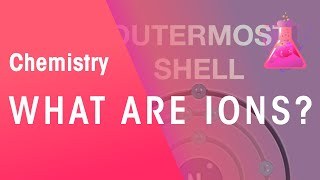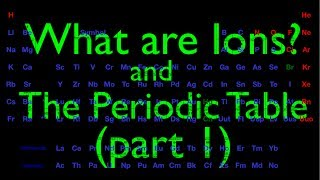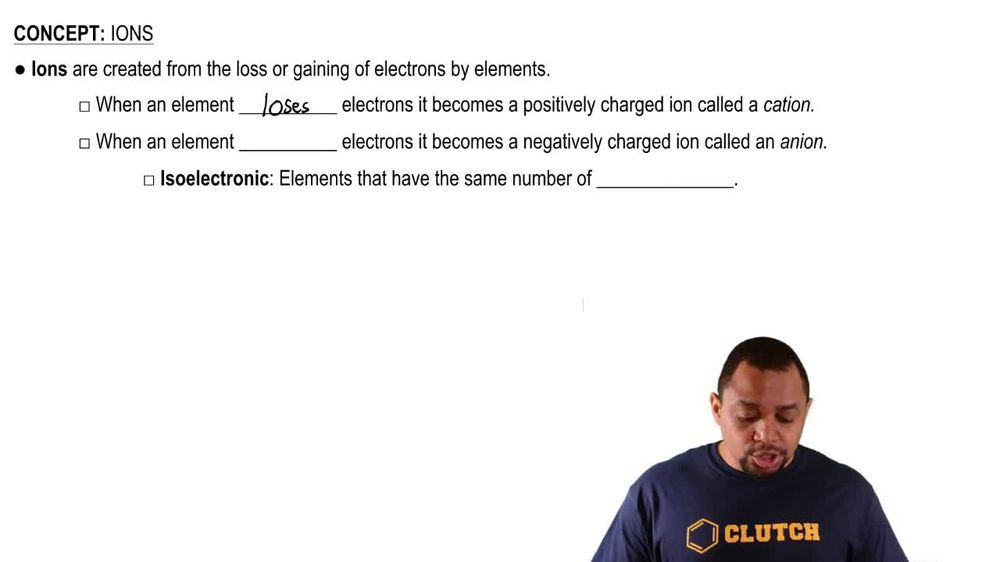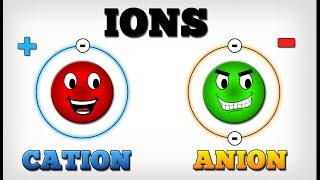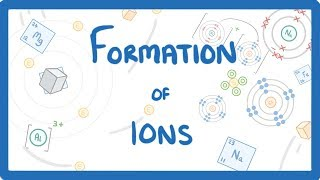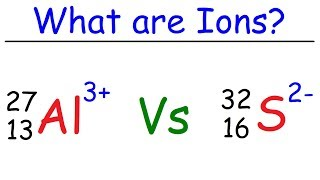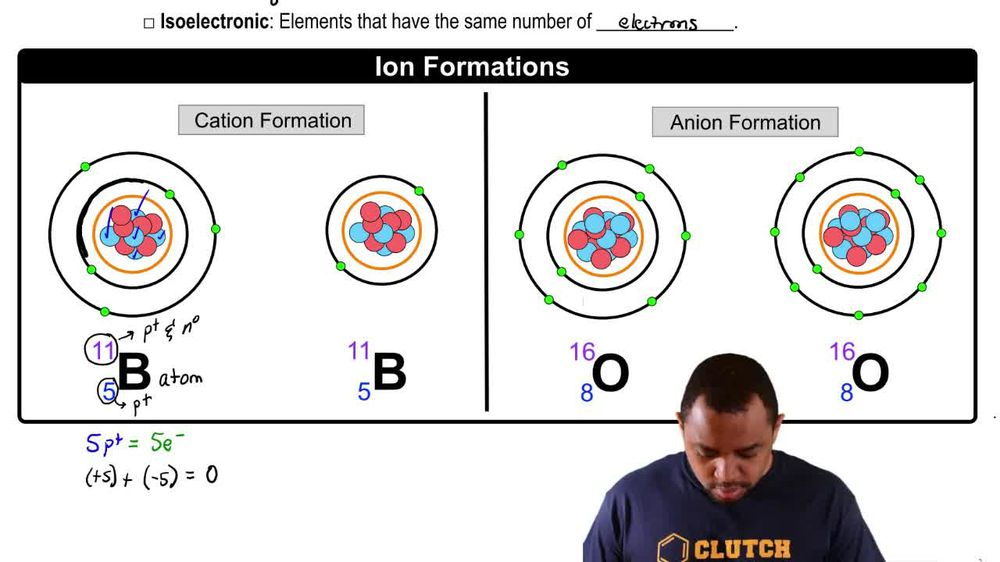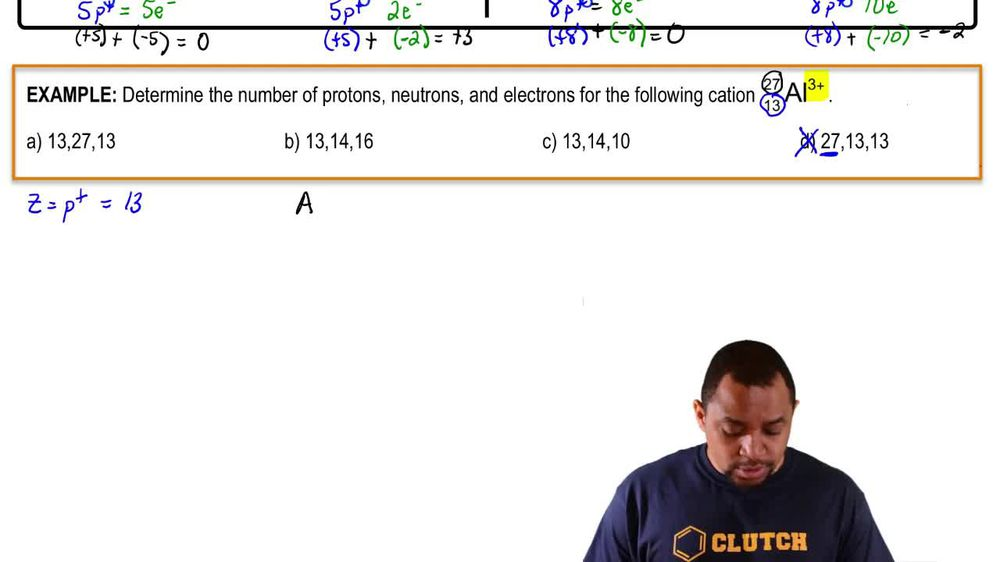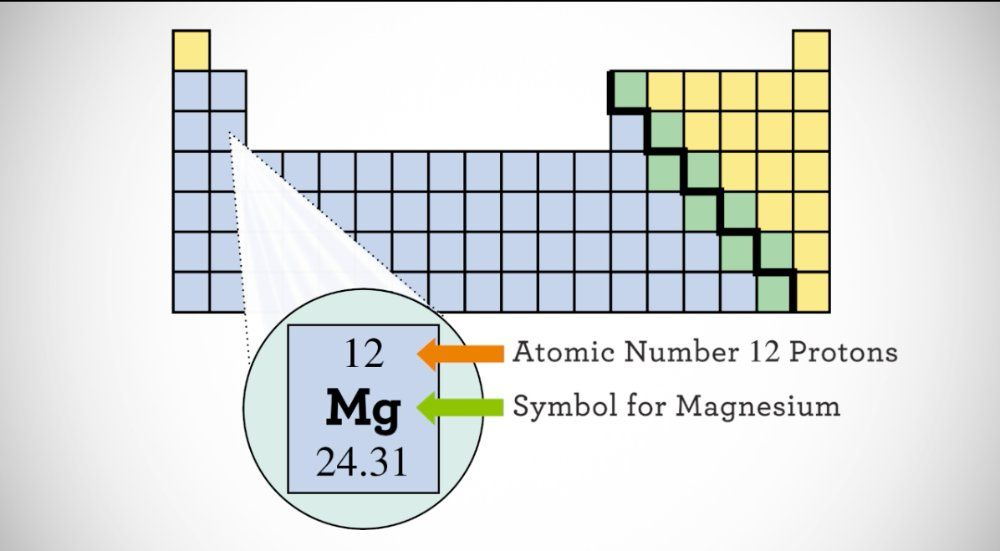Start typing, then use the up and down arrows to select an option from the list.
1. 2. Atoms & Elements2. Ions
Problem

# Determine the number of protons and the number of electrons in each ion. d. Sr2+

Relevant Solution3m
Play a video:
hey everyone in this example, we need to give the number for each subatomic particle in our given atom here, Magnesium 24. So we want to recall the subatomic particles describe. Our number of electrons are number of neutrons and our number of protons in a given atom. As we can see from the answer choices below. So we want to first focus on this number here. In the left hand exponents of our given atom. It's a 24. We want to recognize that any time we have a number in the left hand exponents of our atom, this number will represent the mass number. We want to recall that the mass number is found from taking our number of protons and adding that to our number of neutrons in our given adam. And in this case for magnesium. So let's go ahead and focus on first finding how many protons we have in our atom of magnesium. And we want to recall that our number of protons should equal our atomic number in our atom. And so we can go ahead and find magnesium on the periodic table. We will find magnesium in group two a on the periodic table. And we see a number above that atom, Which says 12. So we want to recall that the number above our atoms in the periodic table or above are elements in the periodic table is our atomic number. So That is what this number is. And so because we see that it corresponds to atomic number 12, we can say that therefore magnesium has 12 protons. So we have determined our number of protons so far. And we want to recall that in neutral atoms in neutral atoms only our number of protons are equal to our number of electrons. Now in this case do we have a neutral atom? No, we do not. Because we have this two plus charge, we have a charged ion of magnesium. And so we want to recall that when we have a plus charge, we have a caddy on. And we want to recall that Canadians lose electrons. And so in this case because we have a two plus charge, our original 12 electrons in our neutral atom of magnesium would therefore lose two electrons based on that charge. And so losing two electrons, we would subtract two electrons and that would leave us with 10 electrons. So this is determined the number of electrons that we have in this magnesium i on here. And the last step is to find our number of neutrons. And so because we are given the mass number and because we have determined our number of protons, we can find the number of neutrons. So we're going to take our mass number which is 24. And we're going to subtract that from our number of protons, which we found to be 12 protons. And this gives us a difference of 12. And so therefore we can say there are 12 neutrons In our Magnesium two plus cat eye on. And so this is going to complete this example. So all of the subatomic particle amounts we've determined correspond to answer choice C as the final correct answer choice to complete this example. So I hope that everything we reviewed was clear. If you have any questions, please leave them down below, and I will see everyone in the next practice video.